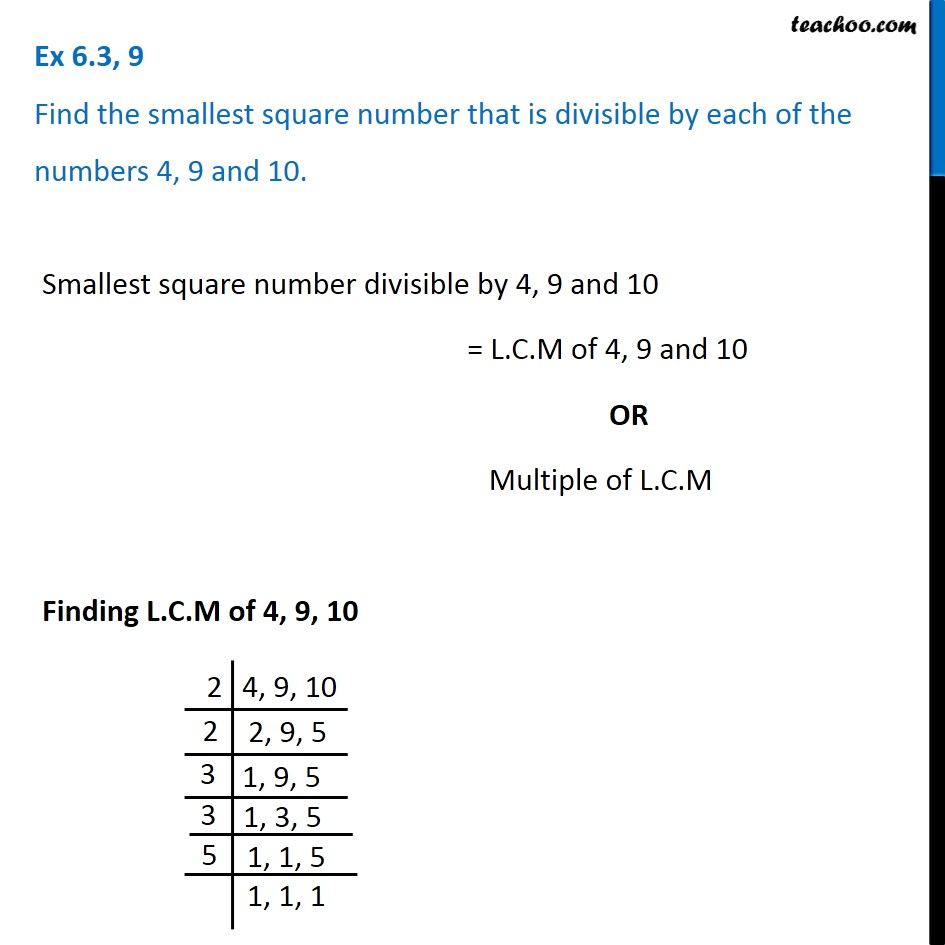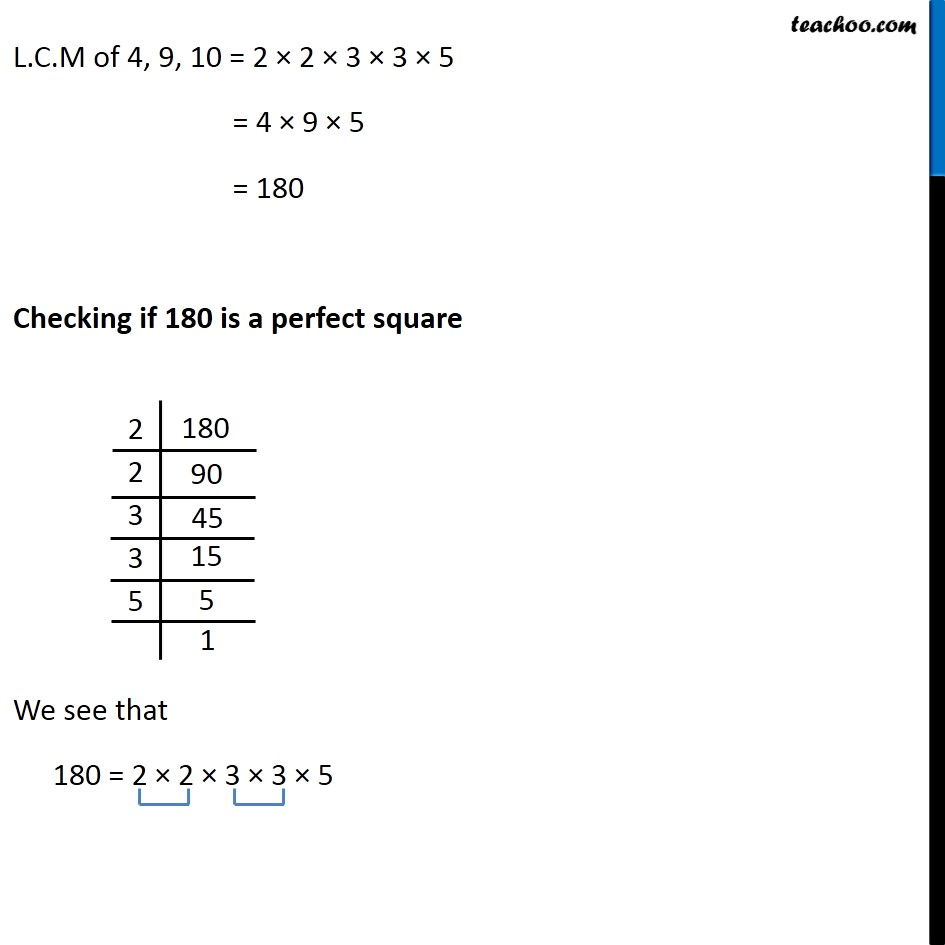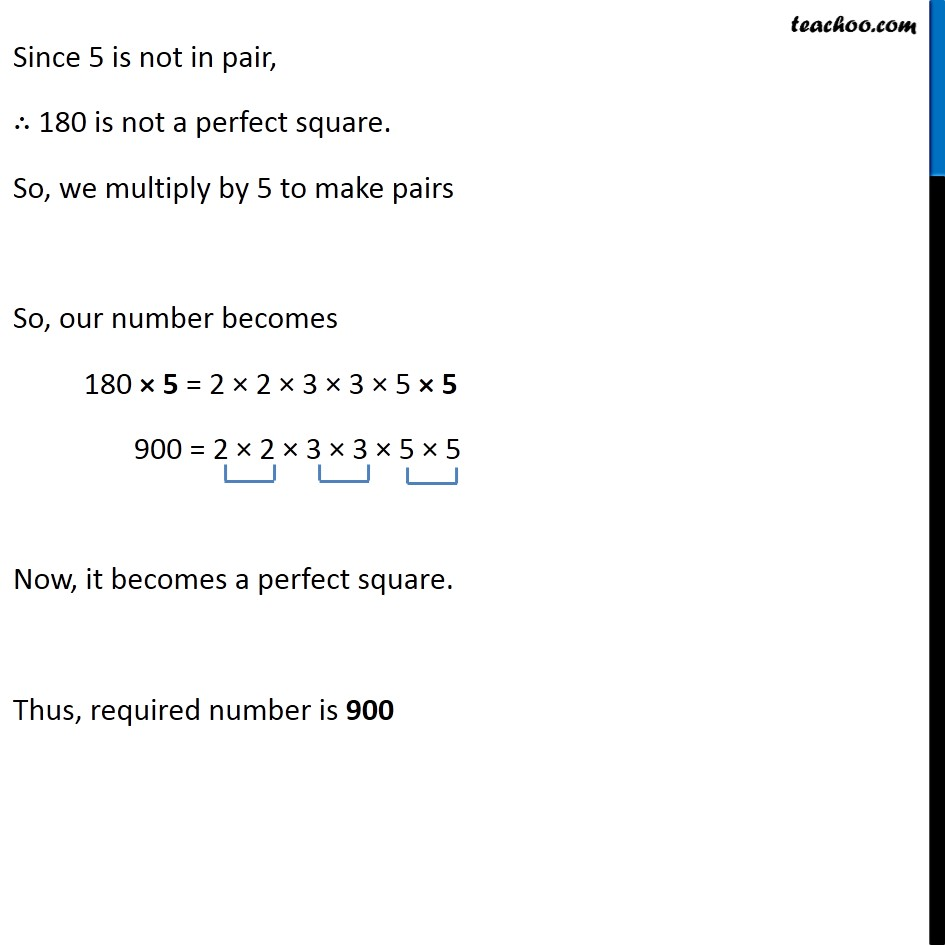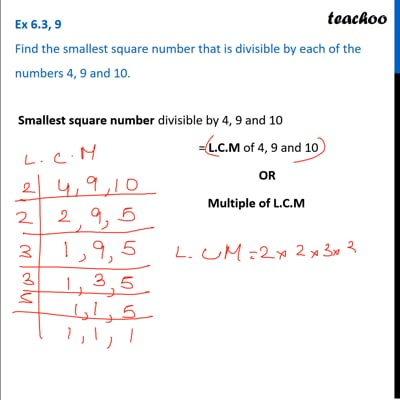Ex 6.3

Chapter 6 Class 8 Squares and Square Roots
Serial order wiseThis video is only available for Teachoo black users

### Transcript

Ex 6.3, 9 Find the smallest square number that is divisible by each of the numbers 4, 9 and 10. Smallest square number divisible by 4, 9 and 10 = L.C.M of 4, 9 and 10 OR Multiple of L.C.M Finding L.C.M of 4, 9, 10 L.C.M of 4, 9, 10 = 2 × 2 × 3 × 3 × 5 = 4 × 9 × 5 = 180 Checking if 180 is a perfect square We see that 180 = 2 × 2 × 3 × 3 × 5 Since 5 is not in pair, ∴ 180 is not a perfect square. So, we multiply by 5 to make pairs So, our number becomes 180 × 5 = 2 × 2 × 3 × 3 × 5 × 5 900 = 2 × 2 × 3 × 3 × 5 × 5 Now, it becomes a perfect square. Thus, required number is 900